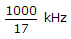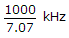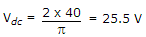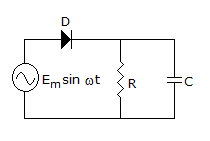# Electronics and Communication Engineering - Analog Electronics

46.

In a capacitor filter, the time constant RLC should be small.

 A. True B. False

Explanation:

Time constant should be large.

47.

A 741 type op-amp has a gain bandwidth product of 1 MHz. A non-inverting amplifier using this op-amp and having a voltage gain of 20 dB will exhibit a -3dB bandwidth of

 A. 50 kHz B. 100 kHz C.D.Explanation:

As we know, Gain Bandwidth, Product = β x A106 = β x A20 log A = 20A = 10'10

β = 100 kHz.

48.

In a full wave rectifier circuit using centre tapped transformer, the peak voltage across half the secondary winding is 40 V. If diodes are ideal, the average output voltage is

 A. 40 V B. 25.5 V C. 20 V D. 2.55 V

Explanation:.

49.

In the circuit of figure, PIV can be up toA. Em B. 2 Em C. 0.5 Em D. 2 Em